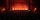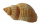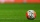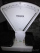# Fraction calculator

The calculator performs basic and advanced operations with fractions, expressions with fractions combined with integers, decimals, and mixed numbers. It also shows detailed step-by-step information about the fraction calculation procedure. Solve problems with two, three, or more fractions and numbers in one expression.

## Result:

### 2/5 + 63/7 - 22/3 = 437/105 = 4 17/105 ≅ 4.1619048

Spelled result in words is four hundred thirty-seven one-hundred fifths (or four and seventeen one-hundred fifths).

### How do you solve fractions step by step?

1. Conversion a mixed number 6 3/7 to a improper fraction: 6 3/7 = 6 3/7 = 6 · 7 + 3/7 = 42 + 3/7 = 45/7

To find new numerator:
a) Multiply the whole number 6 by the denominator 7. Whole number 6 equally 6 * 7/7 = 42/7
b) Add the answer from previous step 42 to the numerator 3. New numerator is 42 + 3 = 45
c) Write a previous answer (new numerator 45) over the denominator 7.

Six and three sevenths is forty-five sevenths
2. Add: 2/5 + 45/7 = 2 · 7/5 · 7 + 45 · 5/7 · 5 = 14/35 + 225/35 = 14 + 225/35 = 239/35
For adding, subtracting, and comparing fractions, it is suitable to adjust both fractions to a common (equal, identical) denominator. The common denominator you can calculate as the least common multiple of both denominators - LCM(5, 7) = 35. In practice, it is enough to find the common denominator (not necessarily the lowest) by multiplying the denominators: 5 × 7 = 35. In the next intermediate step, the fraction result cannot be further simplified by canceling.
In words - two fifths plus forty-five sevenths = two hundred thirty-nine thirty-fifths.
3. Conversion a mixed number 2 2/3 to a improper fraction: 2 2/3 = 2 2/3 = 2 · 3 + 2/3 = 6 + 2/3 = 8/3

To find new numerator:
a) Multiply the whole number 2 by the denominator 3. Whole number 2 equally 2 * 3/3 = 6/3
b) Add the answer from previous step 6 to the numerator 2. New numerator is 6 + 2 = 8
c) Write a previous answer (new numerator 8) over the denominator 3.

Two and two thirds is eight thirds
4. Subtract: the result of step No. 2 - 8/3 = 239/35 - 8/3 = 239 · 3/35 · 3 - 8 · 35/3 · 35 = 717/105 - 280/105 = 717 - 280/105 = 437/105
For adding, subtracting, and comparing fractions, it is suitable to adjust both fractions to a common (equal, identical) denominator. The common denominator you can calculate as the least common multiple of both denominators - LCM(35, 3) = 105. In practice, it is enough to find the common denominator (not necessarily the lowest) by multiplying the denominators: 35 × 3 = 105. In the next intermediate step, the fraction result cannot be further simplified by canceling.
In words - two hundred thirty-nine thirty-fifths minus eight thirds = four hundred thirty-seven one-hundred fifths.

#### Rules for expressions with fractions:

Fractions - use the slash “/” between the numerator and denominator, i.e., for five-hundredths, enter 5/100. If you are using mixed numbers, be sure to leave a single space between the whole and fraction part.
The slash separates the numerator (number above a fraction line) and denominator (number below).

Mixed numerals (mixed fractions or mixed numbers) write as non-zero integer separated by one space and fraction i.e., 1 2/3 (having the same sign). An example of a negative mixed fraction: -5 1/2.
Because slash is both signs for fraction line and division, we recommended use colon (:) as the operator of division fractions i.e., 1/2 : 3.

Decimals (decimal numbers) enter with a decimal point . and they are automatically converted to fractions - i.e. 1.45.

The colon : and slash / is the symbol of division. Can be used to divide mixed numbers 1 2/3 : 4 3/8 or can be used for write complex fractions i.e. 1/2 : 1/3.
An asterisk * or × is the symbol for multiplication.
Plus + is addition, minus sign - is subtraction and ()[] is mathematical parentheses.
The exponentiation/power symbol is ^ - for example: (7/8-4/5)^2 = (7/8-4/5)2

#### Examples:

subtracting fractions: 2/3 - 1/2
multiplying fractions: 7/8 * 3/9
dividing Fractions: 1/2 : 3/4
exponentiation of fraction: 3/5^3
fractional exponents: 16 ^ 1/2
adding fractions and mixed numbers: 8/5 + 6 2/7
dividing integer and fraction: 5 ÷ 1/2
complex fractions: 5/8 : 2 2/3
decimal to fraction: 0.625
Fraction to Decimal: 1/4
Fraction to Percent: 1/8 %
comparing fractions: 1/4 2/3
multiplying a fraction by a whole number: 6 * 3/4
square root of a fraction: sqrt(1/16)
reducing or simplifying the fraction (simplification) - dividing the numerator and denominator of a fraction by the same non-zero number - equivalent fraction: 4/22
expression with brackets: 1/3 * (1/2 - 3 3/8)
compound fraction: 3/4 of 5/7
fractions multiple: 2/3 of 3/5
divide to find the quotient: 3/5 ÷ 2/3

The calculator follows well-known rules for order of operations. The most common mnemonics for remembering this order of operations are:
PEMDAS - Parentheses, Exponents, Multiplication, Division, Addition, Subtraction.
BEDMAS - Brackets, Exponents, Division, Multiplication, Addition, Subtraction
BODMAS - Brackets, Of or Order, Division, Multiplication, Addition, Subtraction.
GEMDAS - Grouping Symbols - brackets (){}, Exponents, Multiplication, Division, Addition, Subtraction.
Be careful, always do multiplication and division before addition and subtraction. Some operators (+ and -) and (* and /) has the same priority and then must evaluate from left to right.

## Fractions in word problems:Add this two mixed numbers: 1 5/6 + 2 2/11=
• ExpressionsLet k represent an unknown number, express the following expressions: 1. The sum of the number n and two 2. The quotient of the numbers n and nine 3. Twice the number n 4. The difference between nine and the number n 5. Nine less than the number n
• A large 2A large popcorn bag holds four times as much as a small popcorn bag at the end of the party 3 1/3 small bags and 2 1/4 large bags left. How many small bags with the leftover popcorn fill?
• Infinite sum of areasAbove the height of the equilateral triangle ABC is constructed an equilateral triangle A1, B1, C1, of the height of the equilateral triangle built A2, B2, C2, and so on. The procedure is repeated continuously. What is the total sum of the areas of all tr
• James 2James collected 24 seashells on the seashore to be shared with his 2 friends. What fractional part of the seashells will each one get if it is distributed evenly? ​
• MarblesDave had 40 marbles. Junjun has 2 1/5 more than Dave’s marbles. How many marbles do they have altogether?Mike buys flowers to plant around his trees. 3/8 of the flowers are red. 1/3 of the flowers are pink. The rest of the flowers are white. Find the fraction of flowers that are white.Sayavong is making cookies for the class. He has a recipe that calls for 3 and 1/2 cups of flour. He has 7/8 of a cup of wheat flour, and 2 and 1/2 cups of white flour. Does Mr. Sayavong have enough flour to make the cookies?Ali bought 5/6 litre of milk. He drank 1/2 litre and his brother drank 1/6 litre. How much litre of milk left?What is 4 1/2+2/7-213/14?Carlos has 4/5 of a tank of fuel in his car. He uses 1/10 of a tank per day. How many days will his fuel last?Rory has 5/8 cup of milk. How much milk does she have left after she doubles the recipe of the smoothie? Berry Smoothie: 2 cups strawberries 1 cup blueberries 1/4 cup milk 1 tbsp (tablespoon) sugar 1/2 tsp (teaspoon) lemon juice 1/8 tsp (teaspoon) vanillaWhat is the sign of expression: minus 18 start fraction 9 divided by 17 end fraction plus left parenthesis minus 18 start fraction 9 divided by 17 end fraction right parenthesis?